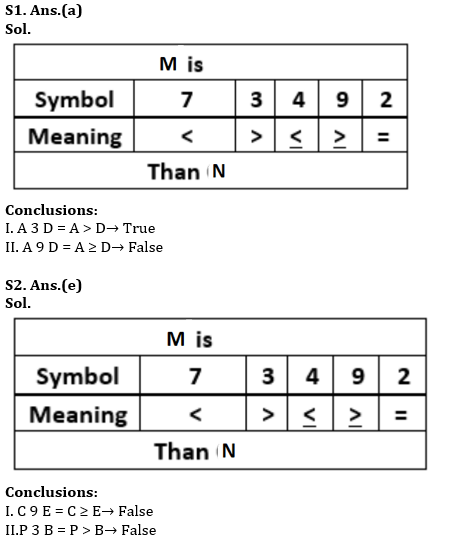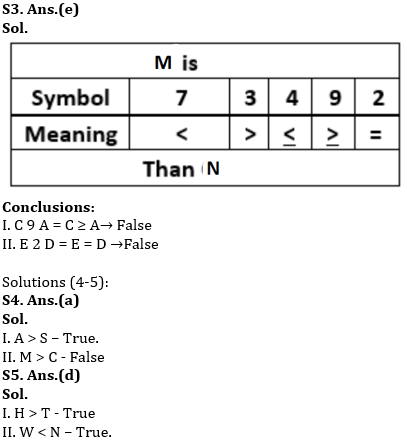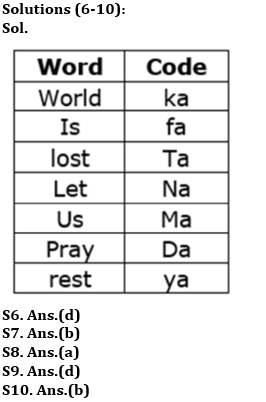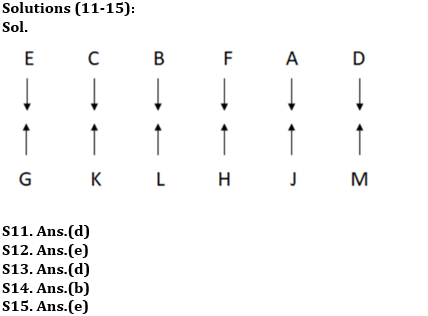Latest Banking jobs   »

# Reasoning Ability Quiz For SIDBI GRADE A 2022- 20th December

Directions (1-3): In the following questions, the symbols 4, 2, 3, 9, and 7 are used with the following meaning as illustrated below:
‘M 4 N’ means ‘M is either smaller than or equal to N’.
‘M 2 N’ means ‘M is neither greater than nor smaller than N’.
‘M 7 N’ means ‘M is neither greater than nor equal to N’.
‘M 3 N’ means ‘M is neither smaller than nor equal to N’
‘M 9 N’ means ‘M is either greater than or equal to N’.
Now in each of the following questions assuming the given statements to be true, find which of the conclusion(s) given below them is/are definitely true?

Q1. Statements: A 3 B 9 C 9 D 3 E, A 3 P 4 Q
Conclusions:
I. A 3 D
II. A 9 D
(a) Only I is true
(b) Both I and II are true
(c) Either I or II is true
(d) Only II is true
(e) Neither I nor II is true

Q2. Statements: A 3 B 9 C 4 D 3 E, A 3 P 4 Q
Conclusions:
I. C 9 E
II. P 3 B
(a) Only I is true
(b) Both I and II are true
(c) Either I or II is true
(d) Only II is true
(e) Neither I nor II is true

Q3. Statements: A 3 B 9 C 4 D 3 E, A 3 P 4 Q
Conclusions:
I. C 9 A
II. E 2 D
(a) Only I is true
(b) Both I and II are true
(c) Either I or II is true
(d) Only II is true
(e) Neither I nor II is true

Directions (4-5): In the following question, some statements are followed by some conclusions. Assuming the given statements to be true, find which of the two conclusions follow(s) the given statements and choose appropriate answer choice.

Q4. Statements:
S < R = C ≤ O = Y, T > O = F > N, A = K = T > M
Conclusions:
I. A > S
II. M > C
(a) Only conclusion I is true
(b) Only conclusion II is true
(c) Either conclusion I or II is true
(d) Both conclusions I and II are true
(e) Neither conclusion I nor II is true

Q5. Statements:
P ≤ U = H < N, P = W ≥ L > T, Q ≤ N = E
Conclusions:
I. H > T
II. W < N
(a) Only conclusion I is true
(b) Only conclusion II is true
(c) Either conclusion I or II is true
(d) Both conclusions I and II are true
(e) Neither conclusion I nor II is true

Directions (6-10): Study the following information carefully and answer the following question.
In a coded language,
‘World is lost’ is coded as ‘ka ta fa’
‘Let us pray’ is coded as ‘da na ma’
‘Lost pray us’ is coded as ‘ta da ma’
‘Us is Rest’ is coded as ‘ya ma fa’

Q6. What is the code of ‘Rest’?
(a) da
(b) fa
(c) ma
(d) ya
(e) Can’t be determined

Q7. What is the code for ‘World pray’?
(a) ka ma
(b) ka da
(c) na ka
(d) sa fa
(e) Can’t be determined

Q8. Which word is coded as ‘Da ta’?
(a) Pray lost
(b) Lost us
(c) Pray me
(d) Is let
(e) Can’t be determined

Q9. What is the code of ‘Let’?
(a) da
(b) fa
(c) ma
(d) na
(e) Can’t be determined

Q10. Which word is coded as ‘fa ma’?
(a) Pray lost
(b) Is us
(c) Pray me
(d) Is let
(e) Can’t be determined

Directions (11-15): Study the following data carefully and answer the questions accordingly.
Twelve people are sitting in two rows. In row 1 – A, B, C, D, E, and F are sitting and facing the south direction and in row 2 – G, H, J, K, L, and M are sitting and facing the north direction but not necessarily in the same order. M sits third to the right of the one, who sits opposite B. E sits second to the right of B, who is a neighbor of F. The one, who sits opposite F, sits two places away from K. H sits to the right of K but not immediately. Less than three people sit between L and G. The one, who sits opposite H, sits two places away from D, who sits near A. G is not a neighbor of H.

Q11. Who sits second to the right of G?
(a) J
(b) The one, who sits near to the one, who sits opposite A.
(c) H
(d)The one, who sits opposite B
(e) None of these

Q12. How many people sit to the left of the one, who sits opposite to L?
(a) One
(b) Two
(c) Four
(d) None
(e) Three

Q13. Who among the following are the immediate neighbors?
(a) E, B
(b) K, J
(c) M, H
(d) G, K
(e) None of these

Q14. How many persons sit between E and the one who sit opposite to M?
(a) One
(b) Four
(c) Three
(d) Two
(e) None

Q15. Find the odd one.
(a) D, M
(b) C, K
(c) F, H
(d) E, G
(e) L, A

Solutions#### Congratulations!Union Budget 2023-24: Free PDF